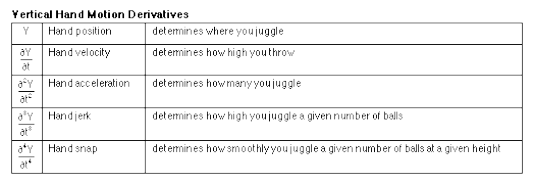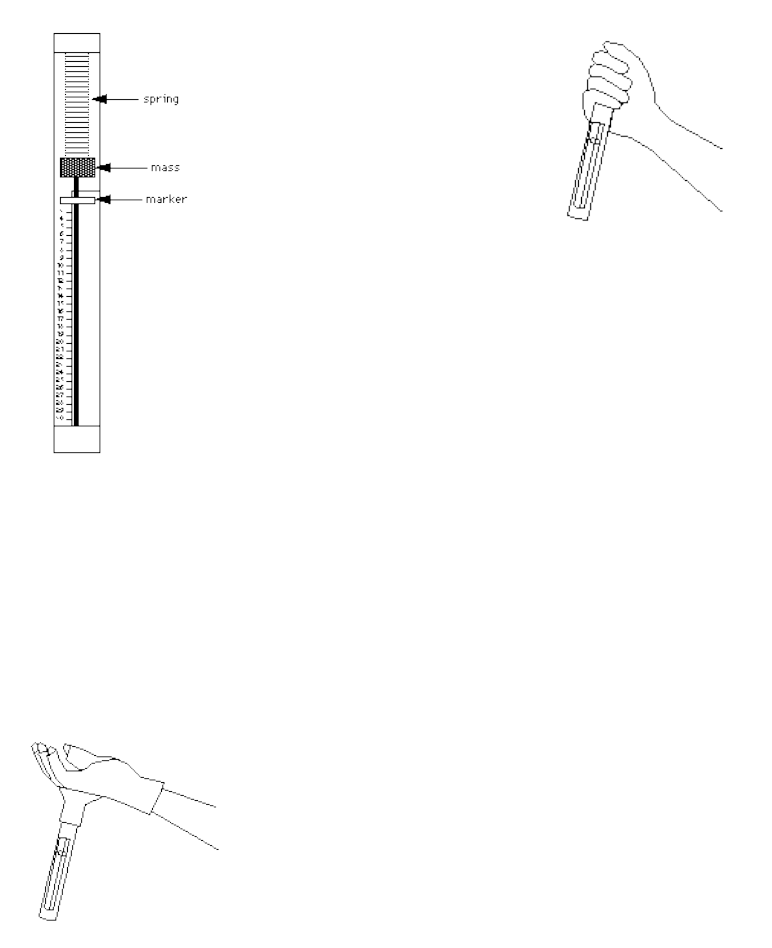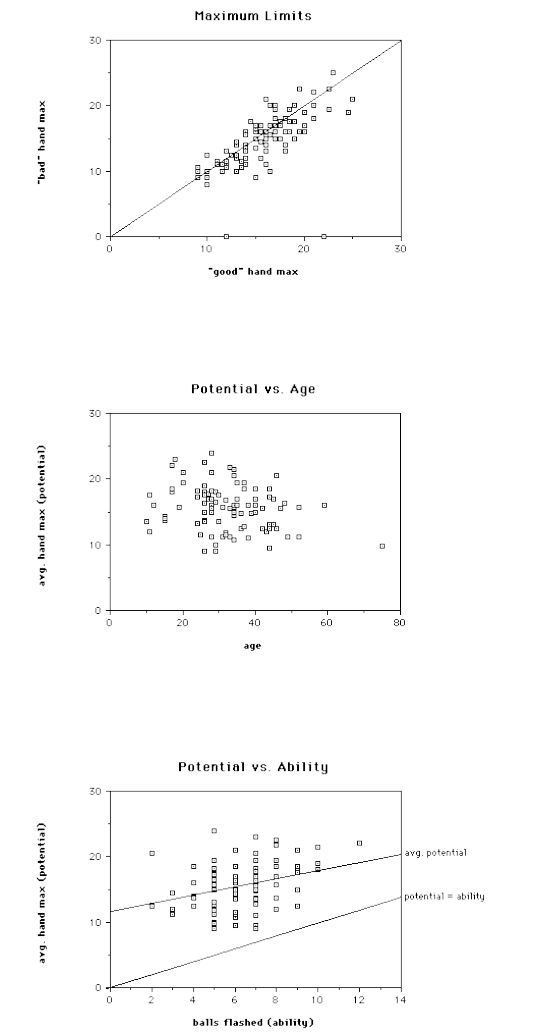In this paper Jack Kalvan, a mechanical engineer and avid juggler, ...
Each hand has $b/h$ objects and so it would take $b/h \tau$ sec...
The equation for the velocity of a ball thrown in the air is $$... In 1997, Kalvan didn't have smartphones or smartwatches equipped wi... Calibration is a matter of getting some experimental points for a l... To illustrate the importance of accuracy when juggling let's calcul...How Many Objects Can Be Juggled Jack Kalvan Originally published in 1997 I hate to break it to you aspiring numbers jugglers, but no human will ever juggle 100 balls. Only a handful of people have reached a level to throw eleven or twelve objects into the air, and so far, not for more than a few seconds. No one has even come close to juggling 13 balls. But is this within the realm of human possibility? Hand speed is one of the main factors that limit the number of objects one can juggle. (The other main fac- tors being accuracy of throws and having long enough arms and enough space in the air for the juggling pat- tern.) I decided to ﬁnd out if anyone has the hand speed necessary to juggle 13 or more balls. So I designed an experiment to measure the theoretical human juggling limits - given the acceleration of the hands. To write the necessary equations, I deﬁne the following variables: b = number of balls h = number of hands f = ﬂight time of a ball from throw to catch τ = time between throws from the same hand V v = vertical throw velocity g = acceleration due to gravity = 9.81 m/s 2 r = "dwell ratio" or fraction of time a hand is holding a ball. My tests show r is usually about 2/3. ω = average number of balls in ﬂight per arc One can also think of r as the average number of balls in a hand while juggling. omega can be expressed as the number of balls per hand minus the balls held in the hand: ω = (b/h) r . ω is also equal to the time that balls are in ﬂight di- vided by how often they are thrown: ω = f . To simplify my analysis, I will assume balls are thrown and caught at the same height. Newtonian physics tells us the ﬂight time of a ball, f = 2V v /g . Substituting this equations for f into the second equation for omega gives ω = 2V v /g. Since g is a constant, we see that omega is proportional to the throwing velocity of the hand divided by the time between throws. This means the number of balls in the air while juggling is closely related to the acceleration of the hand. Although a juggler’s hands do not necessarily accelerate smoothly, the number of balls one can get into the air is approximately proportional to the maximum acceleration of one’s hands . I ﬁgured if I measure the maximum acceleration of a juggler’s hands with a simple accelerometer, I could roughly calculate the juggler’s maximum value for omega. And substituting this value into the equation, b/h = ω + r, gives an approximation of the maximum num- ber of balls one can theoretically juggle. Remember, this maximum number of balls is calculated only from the speed a juggler can potentially throw balls into the air. It does not take into account accuracy of throws or the possibility of collisions. Since the number of balls juggled is proportional to hand acceleration, a corollary is that the height of your juggling pattern is not related to your hand acceleration. For example, if you juggle 5 balls high, you have about the same hand acceleration as if you juggle them low. The diﬀerence is that to juggle high, you accelerate for a longer time and therefore have longer hand motion and a higher throwing velocity. I believe the following chart describes how the deriva- tives of the vertical hand motion relate to juggling: I. THE JUGGLEMETER This simple device measures the hand acceleration. A small mass is connected to a spring inside a tube. When the hand accelerates the device (by shaking it up and down), two opposing forces act on the mass: the acceleration force (force = mass × acceleration) and the spring force (force = spring stiﬀness × distance stretched). These forces are in equilibrium when the spring is stretched. A marker measures the maximum distance the spring was stretched. Since the mass and spring stiﬀness are constant, the maximum acceleration is proportional to this distance. The distance the spring2 stretches is therefore proportional to the number of balls potentially juggled. II. JUGGLEMETER CALIBRATION To calibrate the device, I attached it to the back of a glove. This method worked well for low numbers of balls but the glove and the weight of the jugglemeter made juggling more diﬃcult. Also imperfect juggling led to artiﬁcially high readings because more acceleration is needed to catch inaccurate throws. To calibrate and test the jugglemeter for higher num- bers of balls, I found it was easiest to ﬁrst juggle a number of balls for long enough to get the feel of it. Then, I’d drop the balls, pick up the jugglemeter, and reproduce the same hand motion while holding it. This process is not extremely accurate since it relies on the ability to reproduce the same hand motion as juggling but with the balls removed. However, after tests with many jug- glers and up to 10 balls, and extrapolating the results, I feel conﬁdent that my calibration error is less than 10%. Each juggler I tested repeated the calibration test with an amount of balls that they could juggle comfortably, to convince themselves that the meter calibration was fairly accurate. Some people were unable to simulate the proper hand motion without actually juggling the balls. On the other hand, many were able to reproduce the proper hand motion, and after pretending to juggle 5 balls, were shocked to see the meter reading "5". "How does it know?!!" To ﬁnd the maximum potential of each subject, I told them to shake the jugglemeter as hard and fast as they could, for a few seconds, in an up-and-down motion. (This was the most interesting part for spec- tators.) I measured the maximum with each hand and allowed one retry if they thought they could do better. III. SUMMARY OF TEST RESULTS I tested over 100 people ranging from non-jugglers to some of the best jugglers in the world. Every one of the test subjects had the hand speed to juggle 9 balls. The average was about 16 balls, and the highest recorded was about 24 balls. Hand speed did tend to increase slightly with juggling ability. Those who said they had ﬂashed 9 or more balls averaged about 18.3, while those who had never ﬂashed 5 balls averaged about 14.4. Comparing subjects with comparable juggling ability: hand speed averaged highest for people around 18 years old; there was a small decrease in hand speed with age; and males tended to get slightly higher readings than females. Often there was a diﬀerence between the two hands and many were surprised to ﬁnd they did better with their "bad" hand. I suspect that some of the test subjects were not3 trying as hard as they could have. Also I suspect some could have done better by relaxing their arms more it helped me. IV. CONCLUSION Every person I tested demonstrated the hand acceler- ation needed to juggle a smooth 9 ball cascade. Unfor- tunately, catching wild throws in a messy, inaccurate 9 ball cascade requires much more acceleration than this. And very few of the test subjects have the accuracy to juggle 9 balls smoothly. By practicing, one’s accuracy im- proves, the demands on one’s acceleration are lessened, and the juggling actually requires less and less eﬀort. It requires much less eﬀort to juggle 7 balls well than to juggle 7 balls badly. Here are some illustrations of how inaccuracy leads to higher acceleration. If one makes a throw too far across and has to move the catching hand twice the usual horizontal distance in the same amount of time, this requires twice as much horizontal acceleration. If one’s inaccuracy is in throw height, there is an even more unfortunate relationship. Suppose one hand makes a slightly high throw followed by a slightly low throw, so that the two balls come down at almost the same time. If a throw has to be made in half the normal time, this requires four times the vertical acceleration. If juggling 7 balls, this correction would probably be beyond human capabilities. I believe that eventually someone will juggle 13 balls, and ﬂashing 15 doesn’t seem too unlikely. But how can anyone get to juggling 13 balls smoothly without wasting many years trying to juggle 13 balls badly? It will probably require new training techniques; for exam- ple a technique that allows the juggler to practice throw- ing balls accurately at 15 ball juggling speed, without worrying about catching them and picking them up ev- ery few seconds. But that’s another paper. The equation for the velocity of a ball thrown in the air is$$ v(t) = V_v - gt $$At the point of maximum height, which happens at t=f/2, the velocity of the ball vanishes and so f=2V_v/g. Calibration is a matter of getting some experimental points for a low number of balls and fitting the function y = ax + b, where y is the number of balls per hand, x is the hand acceleration and a and b are constants that are independent from the number of balls being juggled (note that the "dwell ratio" seems to be a characteristic of the juggler and independent of the number of balls). In 1997, Kalvan didn't have smartphones or smartwatches equipped with powerful accelerometers but if you want to determine how many balls you could theoretically juggle based on your hands' acceleration you just need to download [an app like NCSU MyTech](https://projects.delta.ncsu.edu/mytech/). After you download the app simply grab your phone and move it as fast as you can in a juggle like movement. You can then use the data and calculate the maximum number of balls you could juggle - b/h To illustrate the importance of accuracy when juggling let's calculate the horizontal displacement of a ball - \Delta s - caused by a deviation of 1 degree (\Delta \theta) in the throwing angle. ![](https://i.imgur.com/TKWAePh.png) The equations of motion for a ball are$$ y(t) = v_{0y}t-\frac{1}{2}gt^2 \\ v_y = v_{0y}-gt $$Now at the maximum height - h - which happens at time f/2 v_{0y}=g\frac{f}{2} and h = \frac{gf^2}{4}-\frac{gf^2}{8} = \frac{1}{8}gf^2 Now the horizontal position of the ball s is s = fv_{0x} = fv_{0y}\tan \theta = 4h \tan \theta since v_{0x}/v_{0y} = \tan \theta and so \theta = \arctan (s/4h) Now \theta + \Delta \theta = \arctan [(s+\Delta s)/4h], giving \theta = \arctan [(s+\Delta s)/4h] - \arctan (s/4h) Since \arctan(x) = x + O(x^3) we have$$ \Delta \theta \approx \frac{s+\Delta s}{4h}- \frac{s}{4h} = \frac{\Delta s}{4h} $$For h=3m, \Delta \theta = \frac{2 \pi}{360}, \Delta s is 21 cm (!), which means that if you want to attempt to juggle a lot of balls hand acceleration is important but you also need an incredible throwing precision. In this paper Jack Kalvan, a mechanical engineer and avid juggler, tries to answer the following question: What is the maximum number of objects a human can juggle? The analysis is centered around the maximum velocity of the hands, which being a limiting factor, is only one piece of a more complex puzzle that involves throwing accuracy among others. Kalvan writes that flashing 15 balls (each ball is only thrown and caught once) "doesn't seem too unlikely" and his prediction appears to be not too far from reality - in April 2017, Alex Barron was able to flash 14 balls. [!['aw'](https://i.ytimg.com/vi/yWO-_b8JO3A/maxresdefault.jpg)](https://www.youtube.com/watch?v=WK6AlhTjUbY) [For more information on Alex's record you can check this [article](https://www.wired.com/story/the-physicsand-physicalityof-extreme-juggling/)] Each hand has b/h objects and so it would take b/h \tau seconds for a hand to go through a full cycle. Now, since each object will spend a fraction of time in the hand (r \tau), the total time each object spends in the air is simply the subtraction of these 2 times: f = b/h \tau - r \tau or equivalently f/\tau = b/h - r. Now, at the point at which the objects reach the hand, the flux of incoming objects has to be equal to the flux of outgoing objects, otherwise there's going to be either a shortage or an accumulation of objects and the juggling pattern will break. ![](https://i.imgur.com/E6qJSH1.png) Since objects are being thrown in the air at a frequency of 1/\tau and new objects are arriving at a frequency of 1/f times the average number of objects in the air (\omega) we get$$ \frac{\omega}{f} = \frac{1}{\tau} $$or$$ \omega = \frac{f}{\tau} $$Joining the two expressions we have$$ \omega= b/h - r